﻿

### A structure theorem for echelon Köthe spaces

#### Abstract

In , Lopez-Molina defined the echelon Köthe spaces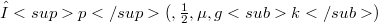$Λp(\Chi,𝛽,\mu,gk)$, which provide a suitable generalization of the echelon sequence spaces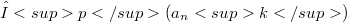$λp(a_{n}k)$ to a general measure space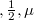$\Chi, 𝛽, \mu$ (see also ). In this paper, we show that the strutture of the separable echelon Köthe spaces is «nicely» close to the strutture of the echelon sequence spaces. Namely, our main result is: Let$Λp(\Chi,𝛽,\mu,gk)$ be a separable echelon Köthe space of order p,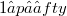$1 ≤ p ≤ ∈fty$, with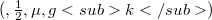$(\Chi,𝛽,\mu,gk)$ purely non-atomic. Then, there is an echelon sequence space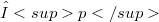$λp$ so that$Λp$ is isomorphic to the space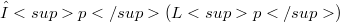$λp(Lp)$ of all$λp$-summable sequences in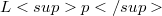$Lp$. An an application we show that a separable echelon Köthe space has a basis (by a basis we mean a Schauder basis), which is unconditional if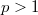$p > 1$.

DOI Code: 10.1285/i15900932v9n2p165

Full Text: PDF